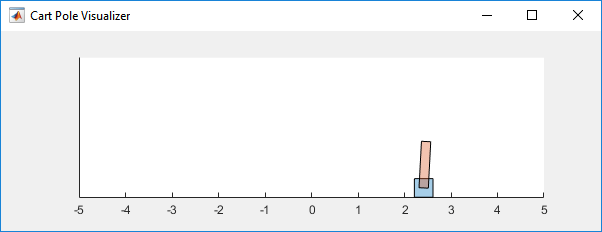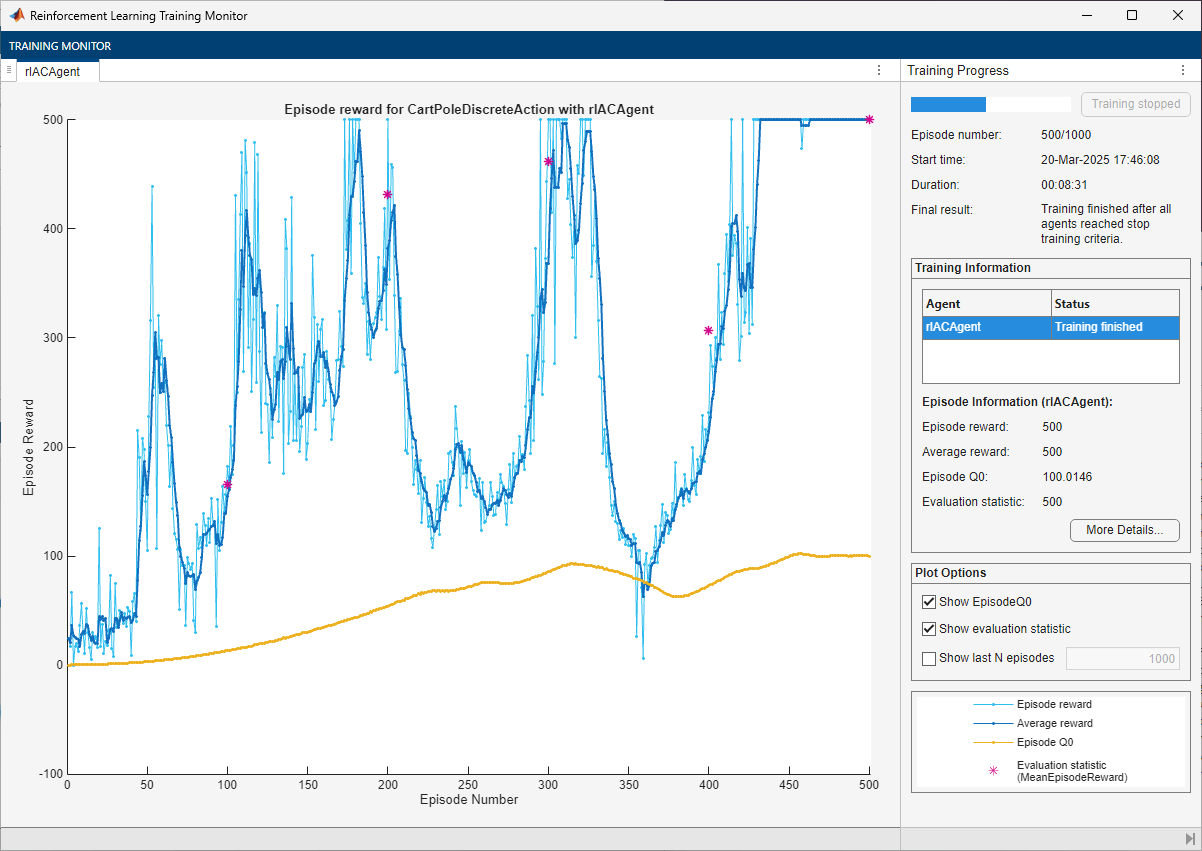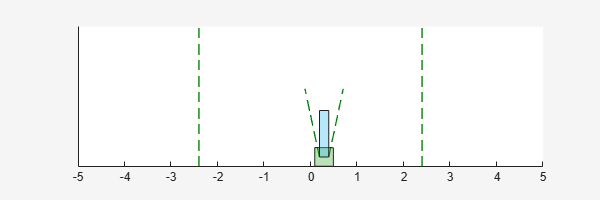# Train AC Agent to Balance Cart-Pole System

This example shows how to train an actor-critic (AC) agent to balance a cart-pole system modeled in MATLAB®.

For more information on AC agents, see Actor-Critic (AC) Agents. For an example showing how to train an AC agent using parallel computing, see Train AC Agent to Balance Cart-Pole System Using Parallel Computing.

### Cart-Pole MATLAB Environment

The reinforcement learning environment for this example is a pole attached to an unactuated joint on a cart, which moves along a frictionless track. The training goal is to make the pendulum stand upright without falling over.For this environment:

• The upward balanced pendulum position is `0` radians, and the downward hanging position is `pi` radians.

• The pendulum starts upright with an initial angle between –0.05 and 0.05 rad.

• The force action signal from the agent to the environment is either –10 or 10 N.

• The observations from the environment are the position and velocity of the cart, the pendulum angle, and the pendulum angle derivative.

• The episode terminates if the pole is more than 12 degrees from vertical or if the cart moves more than 2.4 m from the original position.

• A reward of +1 is provided for every time step that the pole remains upright. A penalty of –5 is applied when the pendulum falls.

### Create Environment Interface

Create a predefined environment interface for the pendulum.

`env = rlPredefinedEnv("CartPole-Discrete")`
```env = CartPoleDiscreteAction with properties: Gravity: 9.8000 MassCart: 1 MassPole: 0.1000 Length: 0.5000 MaxForce: 10 Ts: 0.0200 ThetaThresholdRadians: 0.2094 XThreshold: 2.4000 RewardForNotFalling: 1 PenaltyForFalling: -5 State: [4x1 double] ```
`env.PenaltyForFalling = -10;`

The interface has a discrete action space where the agent can apply one of two possible force values to the cart, –10 or 10 N.

Obtain the observation and action information from the environment interface.

```obsInfo = getObservationInfo(env); actInfo = getActionInfo(env);```

Fix the random generator seed for reproducibility.

`rng(0)`

### Create AC Agent

An AC agent approximates the discounted cumulative long-term reward using a value-function critic. A value-function critic must accept an observation as input and return a single scalar (the estimated discounted cumulative long-term reward) as output.

To approximate the value function within the critic, use a neural network. Define the network as an array of layer objects, and get the dimension of the observation space and the number of possible actions from the environment specification objects. For more information on creating a deep neural network value function representation, see Create Policies and Value Functions.

```criticNet = [ featureInputLayer(obsInfo.Dimension(1)) fullyConnectedLayer(32) reluLayer fullyConnectedLayer(1)];```

Convert to `dlnetwork` and display the number of weights.

```criticNet = dlnetwork(criticNet); summary(criticNet)```
``` Initialized: true Number of learnables: 193 Inputs: 1 'input' 4 features ```

Create the critic approximator object using `criticNet`, and the observation specification. For more information, see `rlValueFunction`.

`critic = rlValueFunction(criticNet,obsInfo);`

Check the critic with a random observation input.

`getValue(critic,{rand(obsInfo.Dimension)})`
```ans = single -0.3590 ```

An AC agent decides which action to take using a stochastic policy, which for discrete action spaces is approximated by a discrete categorical actor. This actor must take the observation signal as input and return a probability for each action.

To approximate the policy function within the actor, use a deep neural network. Define the network as an array of layer objects, and get the dimension of the observation space and the number of possible actions from the environment specification objects.

```actorNet = [ featureInputLayer(obsInfo.Dimension(1)) fullyConnectedLayer(32) reluLayer fullyConnectedLayer(numel(actInfo.Elements)) softmaxLayer]; ```

Convert to `dlnetwork` and display the number of weights.

```actorNet = dlnetwork(actorNet); summary(actorNet)```
``` Initialized: true Number of learnables: 226 Inputs: 1 'input' 4 features ```

Create the actor approximator object using `actorNet` and the observation and action specifications. For more information, see `rlDiscreteCategoricalActor`.

`actor = rlDiscreteCategoricalActor(actorNet,obsInfo,actInfo);`

To return the probability distribution of the possible actions as a function of a random observation, and given the current network weights, use evaluate.

`prb = evaluate(actor,{rand(obsInfo.Dimension)})`
```prb = 1x1 cell array {2x1 single} ```
`prb{1}`
```ans = 2x1 single column vector 0.4414 0.5586 ```

Create the agent using the actor and critic. For more information, see `rlACAgent`.

`agent = rlACAgent(actor,critic);`

Check the agent with a random observation input.

`getAction(agent,{rand(obsInfo.Dimension)})`
```ans = 1x1 cell array {[-10]} ```

Specify agent options, including training options for the actor and critic, using dot notation. Alternatively, you can use `rlACAgentOptions` and `rlOptimizerOptions` objects before creating the agent.

```agent.AgentOptions.EntropyLossWeight = 0.01; agent.AgentOptions.ActorOptimizerOptions.LearnRate = 1e-2; agent.AgentOptions.ActorOptimizerOptions.GradientThreshold = 1; agent.AgentOptions.CriticOptimizerOptions.LearnRate = 1e-2; agent.AgentOptions.CriticOptimizerOptions.GradientThreshold = 1;```

### Train Agent

To train the agent, first specify the training options. For this example, use the following options.

• Run each training episode for at most 1000 episodes, with each episode lasting at most 500 time steps.

• Display the training progress in the Episode Manager dialog box (set the `Plots` option) and disable the command line display (set the `Verbose` option to `false`).

• Stop training when the agent receives an average cumulative reward greater than 480 over 10 consecutive episodes. At this point, the agent can balance the pendulum in the upright position.

For more information, see `rlTrainingOptions`.

```trainOpts = rlTrainingOptions(... MaxEpisodes=1000,... MaxStepsPerEpisode=500,... Verbose=false,... Plots="training-progress",... StopTrainingCriteria="AverageReward",... StopTrainingValue=480,... ScoreAveragingWindowLength=10);```

You can visualize the cart-pole system during training or simulation using the `plot` function.

`plot(env)`Train the agent using the `train` function. Training this agent is a computationally intensive process that takes several minutes to complete. To save time while running this example, load a pretrained agent by setting `doTraining` to `false`. To train the agent yourself, set `doTraining` to `true`.

```doTraining = false; if doTraining % Train the agent. trainingStats = train(agent,env,trainOpts); else % Load the pretrained agent for the example. load("MATLABCartpoleAC.mat","agent"); end ```### Simulate AC Agent

To validate the performance of the trained agent, simulate it within the cart-pole environment. For more information on agent simulation, see `rlSimulationOptions` and `sim`.

```simOptions = rlSimulationOptions(MaxSteps=500); experience = sim(env,agent,simOptions);````totalReward = sum(experience.Reward)`
```totalReward = 500 ```